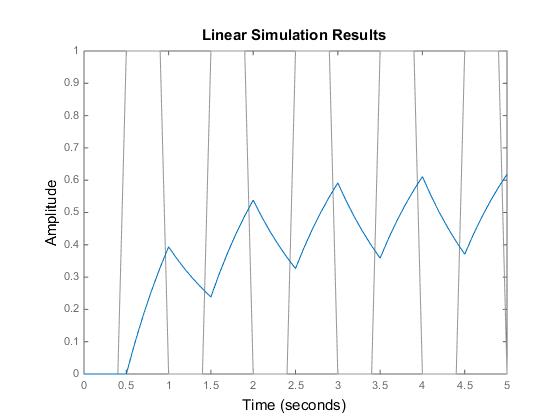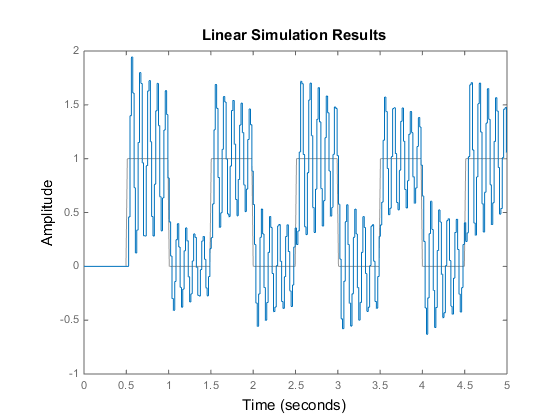# lsim

Simulate time response of dynamic system to arbitrary inputs

## Syntax

```lsim(sys,u,t) lsim(sys,u,t,x0) lsim(sys,u,t,x0,method) lsim(sys1,...,sysn,u,t) lsim(sys1,LineSpec1,...,sysN,LineSpecN,u,t) y = lsim(___) [y,t,x] = lsim(___) lsim(sys) ```

## Description

`lsim` simulates the (time) response of continuous or discrete linear systems to arbitrary inputs. When invoked without left-hand arguments, `lsim` plots the response on the screen.

`lsim(sys,u,t)` produces a plot of the time response of the dynamic system model `sys` to the input history, `t`,`u`. The vector `t` specifies the time samples for the simulation (in system time units, specified in the `TimeUnit` property of `sys`), and consists of regularly spaced time samples:

```t = 0:dt:Tfinal ```

The input `u` is an array having as many rows as time samples (`length(t)`) and as many columns as system inputs. For instance, if `sys` is a SISO system, then `u` is a t-by-1 vector. If `sys` has three inputs, then `u` is a t-by-3 array. Each row `u(i,:)` specifies the input value(s) at the time sample `t(i)`. The signal `u` also appears on the plot.

The model `sys` can be continuous or discrete, SISO or MIMO. In discrete time, `u` must be sampled at the same rate as the system. In this case, the input `t` is redundant and can be omitted or set to an empty matrix. In continuous time, the time sampling `dt = t(2)-t(1)` is used to discretize the continuous model. If `dt` is too large (undersampling), `lsim` issues a warning suggesting that you use a more appropriate sample time, but will use the specified sample time. See Algorithms for a discussion of sample times.

`lsim(sys,u,t,x0) ` further specifies an initial condition `x0` for the system states. This syntax applies only when `sys` is a state-space model. `x0` is a vector whose entries are the initial values of the corresponding states of `sys`.

`lsim(sys,u,t,x0,method)` explicitly specifies how the input values should be interpolated between samples, when `sys` is a continuous-time system. Specify `method` as one of the following values:

• `'zoh'` — Use zero-order hold

• `'foh'` — Use linear interpolation (first-order hold)

If you do not specify a method, `lsim` selects the interpolation method automatically based on the smoothness of the signal `u`.

`lsim(sys1,...,sysn,u,t)` simulates the responses of several dynamic system models to the same input history `t`,`u` and plots these responses on a single figure. You can also use the `x0` and `method` input arguments when computing the responses of multiple models.

`lsim(sys1,LineSpec1,...,sysN,LineSpecN,u,t)` specifies the line style, marker, and color of each of the system responses in the plot. You can also use the `x0` and `method` input arguments with this syntax. Each `LineSpec` argument is specified as a vector of one, two, or three characters. The characters can appear in any order. For example, the following code plots the response of `sys1` as a yellow dotted line and the response of `sys2` as a green dashed line:

```lsim(sys1,'y:',sys2,'g--',u,t,x0) ```

For more information about configuring this argument, see the `LineSpec` input argument of the `plot` function.

`y = lsim(___)` returns the system response `y`, sampled at the same times as the input (`t`). The output `y` is an array having as many rows as time samples (`length(t)`) and as many columns as system outputs. No plot is drawn on the screen. You can use this syntax with any of the input arguments described in previous syntaxes except the `LineSpec` arguments.

`[y,t,x] = lsim(___)` also returns the time vector `t` used for simulation and the state trajectories `x` (for state-space models only). The output `x` has as many rows as time samples (`length(t)`) and as many columns as system states. You can use this syntax with any of the input arguments described in previous syntaxes except the `LineSpec` arguments.

`lsim(sys)` opens the Linear Simulation Tool GUI. For more information about working with this GUI, see Working with the Linear Simulation Tool.

## Examples

collapse all

Simulate and plot the response of the following system to a square wave with period of four seconds:

`$H\left(s\right)=\left[\begin{array}{c}\frac{2{s}^{2}+5s+1}{{s}^{2}+2s+3}\\ \frac{s-1}{{s}^{2}+s+5}\end{array}\right].$`

Create the transfer function, and generate the square wave with `gensig`. Sample every 0.1 second during 10 seconds.

```H = [tf([2 5 1],[1 2 3]);tf([1 -1],[1 1 5])]; [u,t] = gensig('square',4,10,0.1);```

Then simulate with `lsim`.

`lsim(H,u,t)`The plot displays both the applied signal and the response.

Load estimation data to estimate a model.

```load(fullfile(matlabroot,'toolbox','ident','iddemos','data','dcmotordata')); z = iddata(y,u,0.1,'Name','DC-motor');```

`z` is an `iddata` object that stores the 1-input 2-output estimation data with sample time 0.1 seconds.

Estimate a state-space model of order 4 using estimation data `z`.

`[sys,x0] = n4sid(z,4);`

`sys` is the estimated model and `x0` are the estimated initial states.

Simulate the response of `sys` using the same input data as the one used for estimation and the initial states returned by the estimation command.

`[y,t,x] = lsim(sys,z.InputData,[],x0);`

Here, `y` is the system response, `t` is the time vector used for simulation, and `x` is the state trajectory.

Compare the simulated response `y` to measured response `z.OutputData` for both outputs.

```subplot(211), plot(t,z.OutputData(:,1),'k',t,y(:,1),'r') legend('Measured','Simulated') subplot(212), plot(t,z.OutputData(:,2),'k',t,y(:,2),'r') legend('Measured','Simulated')```## Algorithms

Discrete-time systems are simulated with `ltitr` (state space) or `filter` (transfer function and zero-pole-gain).

Continuous-time systems are discretized with `c2d` using either the `'zoh'` or `'foh'` method (`'foh'` is used for smooth input signals and `'zoh'` for discontinuous signals such as pulses or square waves). The sample time is set to the spacing `dt` between the user-supplied time samples `t`.

The choice of sample time can drastically affect simulation results. To illustrate why, consider the second-order model

`$\begin{array}{cc}H\left(s\right)=\frac{{\omega }^{2}}{{s}^{2}+2s+{\omega }^{2}},& \omega =62.83\end{array}$`

To simulate its response to a square wave with period 1 second, you can proceed as follows:

```w2 = 62.83^2; h = tf(w2,[1 2 w2]); t = 0:0.1:5; % vector of time samples u = (rem(t,1) >= 0.5); % square wave values lsim(h,u,t) ```

`lsim` evaluates the specified sample time, and issues a warning:

```Warning: Input signal is undersampled. Sample every 0.016 sec or faster. ```To improve on this response, discretize H(s) using the recommended sample time:

```dt = 0.016; ts = 0:dt:5; us = (rem(ts,1) >= 0.5); hd = c2d(h,dt); lsim(hd,us,ts) ```This response exhibits strong oscillatory behavior that is hidden in the undersampled version.